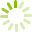# Math 10 Part 2 (MATH 0751)

This course provides students the background foundation in introductory algebra and geometry. It is particularly suitable for students who have not studied algebra before or who need to upgrade before taking Math 11. The algebra section includes: real numbers, equations, word problems, exponents, scientific notation, graphs of linear equations, data analysis, operations with polynomials, and simple factoring. The geometry section includes: a study of plane figures, basic constructions, angle relationships and measurements, parallel lines, congruent and similar triangles, Pythagoras' Theorem, and basic trigonometric ratios. Both MATH 0750 and MATH 0751 are required for completion of ABE Intermediate level Mathematics.
Course code: MATH 0751
Credits: 4.0
Length: 96.0 hours
Course outline: view https://www.vcc.ca/vccphp/courseoutline?subject=MATH&number=0751

## Prerequisites

MATH 0750 with a C-; Math 9 with a C-; Apprenticeship and Workplace Math 10 with a C; or 80% on the Basic Arithmetic Assessment.